takes closest neighbors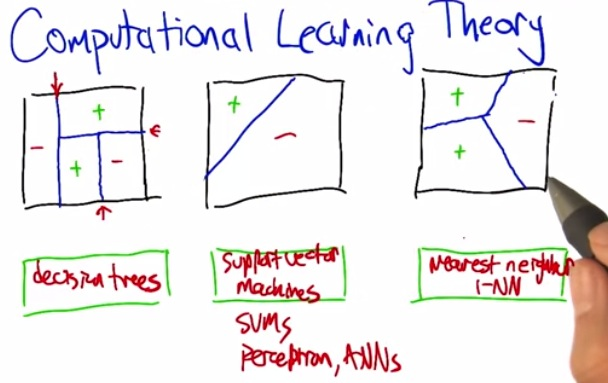• The first one is decision trees. First it split the region 1/3 left, 2/3 right. then make a horizontal split. and finally another vertical split. So it's splitting into two region, on and on.
• The second one is support vector machines. We can see that it's perfectly split the dataset in the middle for both region.
• The final one is nearest neighbors, particularly 1nn. We can see that the same for nearer region over the other.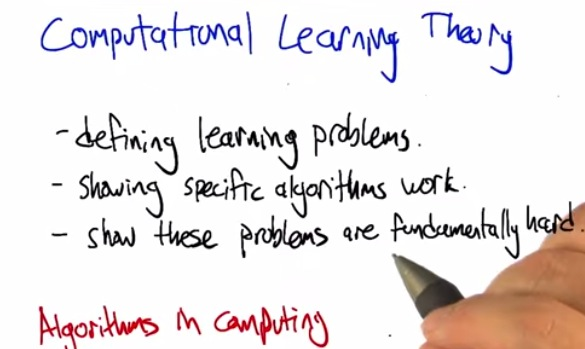• The CLT will help us, what the problems are, what the algorithms work and might not work.
• And also some problems are harder for some algorithms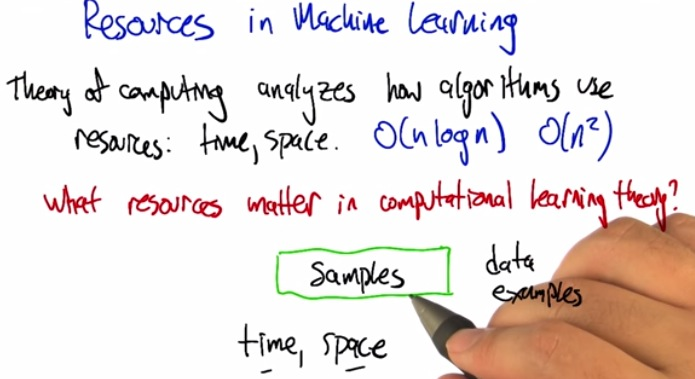• For programming tine and space continuum may be important, but samples are more matters. Because few samples tend to overfit, but more samples can make it more generalize.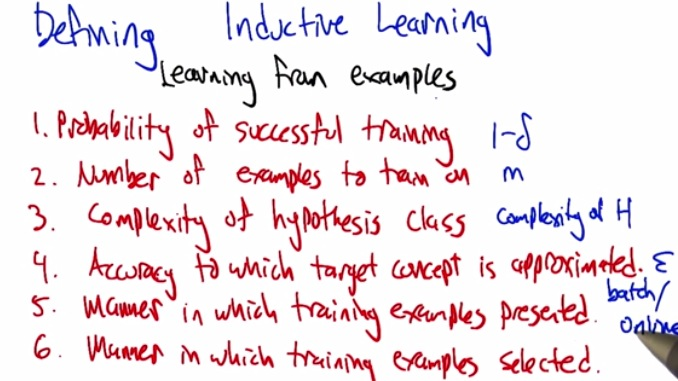• What are the probability that our learning predict accurately. We can measure by the success of it by (1-error rate)
• The number of example
• THe complexity, if it's too simple, then it's bias, can handle for much data. If it's too complex, tend to overfit.
• Is the target have the most generalize function(epsilon)
• How is presented? Batch vs stochastic.
• Next to be discussed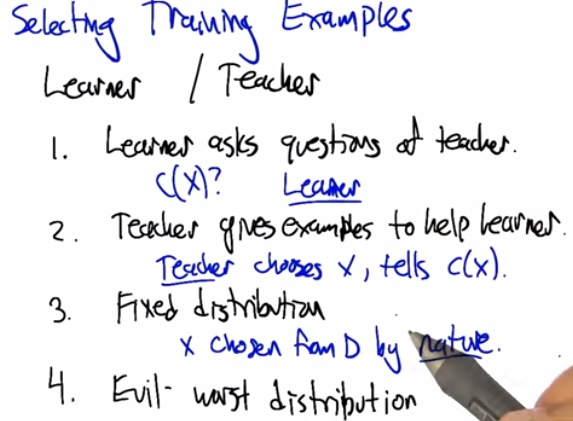• for particular selecting the data, how much data we needed
• For teacher, there's learner in reality.
• One that gives quiz directly, or the teachers that gives examples to solve.
• And there's two distribution of the data.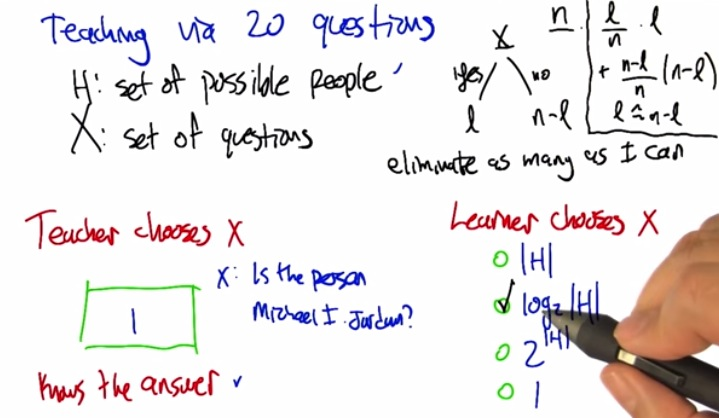• This is the 20 questions earlier.
• If teacher is asked something that he already know (in 20 questions) then he should be able to guess correclty in 1 steps. Something that's called cheating.
• But if learner(machine learning) asked something it doesn't know, then the strategy would be different
• As H is set of possible hypothesis(path), and X is set of questions, than like decision tree, finding the best hypothesis would be logH notation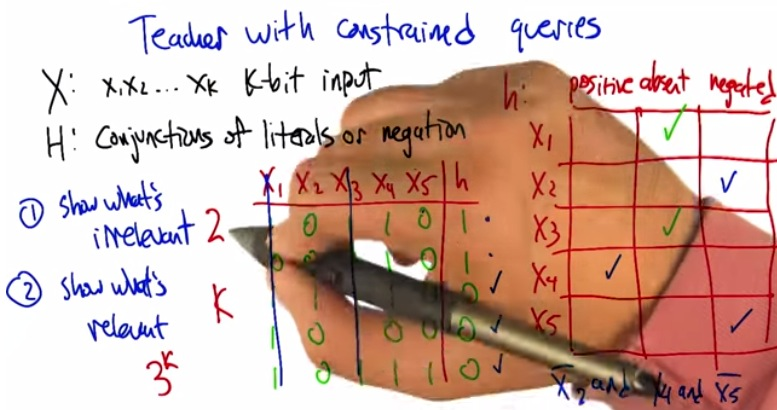• not in reality if we list hypothesis of all possible cases.
• Consider above cases, we infer what the hypothesis are, and build ing all the sample.
• Then on the other case, given following samples, infer what are the hypothesis.
• Good teacher can shows what irrelevant, (either 1 or 0) and shows what relevant 3^k(positive,absent,negated)
• But what if the case that the teacher (us) don't know all good examples(produce 1)?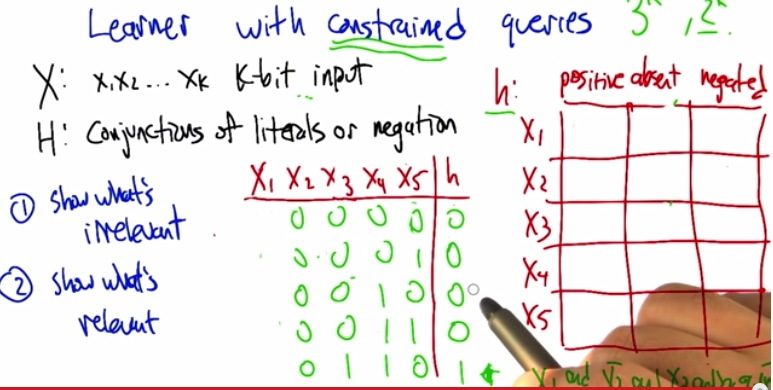• If given best hypothesis, the teacher know that, the learner guessing all the possible answers, in binary it will be 2^k(k = nx). The learner just constrained at the learning table, not guess based on positive/absent/negated, that would mean cheating.
• Then the learner itself wouldn't have log2n steps, (because the teacher doesn't give them a clue until the learner got it), the learner will told all possible combinations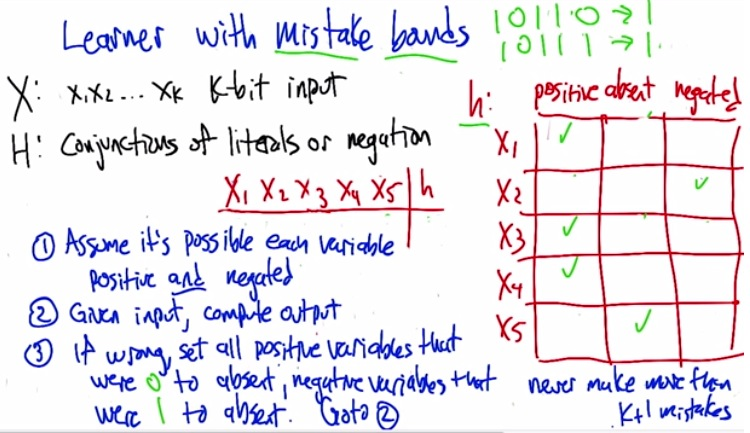• So in this case, the learner now granted the power of mistake bounds.
• For every input arrives, if there's mistake predicting the output, charge it.
• Suppose we set init all positive and negative, and keep receiving the input (follow the algorithm).
• If we get true, then we set it to definite true. the first pattern, will assign all points to not absent, and one get zero, the negated becomes just that. The second one, makes the learner ignore X5 because it doesn't matters
• So we only learn with k+1 examples, that is learn for all possible result positive.